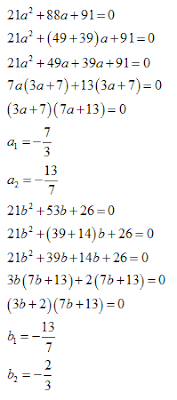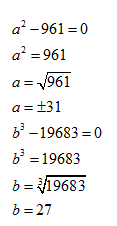# Quadractic Equations For IBPS PO: Part 4

1. In the following question two equations (A) and (B) given. You have to solve both:
I. 21a² + 88a + 91 = 0
II. 21b² + 53b + 26 = 0

A. If a < b
B. If a b
C. If a = b
D. If a > b
E. If a > b

2. In the following question two equations (A) and (B) given. You have to solve both:
I. 5a² - 31a + 44 = 0
II. 5b² +4b -33=0

A. If a
B. If a > b
C. If a = b
D. If a > b
E. If a < b

3. In the following question two equations (A) and (B) given. You have to solve both:
I. a² - 23a + 112 = 0
II. b² + 7b - 78 = 0

A. If a >
B. If a = b
C. If a > b
D. If a < b
E. If a b

4. In the following question two equations (A) and (B) given. You have to solve both:
I. 5a² + 43a - 18 = 0
II. b²- 7b+ 12 =0

A. If a = b
B. If a > b
C. If a < b
D. If a b
E.  If a > b

5. In the following question two equations (A) and (B) given. You have to solve both:
I. a²- 961 = 0
II. b³ - 19863 = 0

A.  If a > b
B. If a < b
C. If a b
D. If a > b
E.  If a = b

## Solution:

#### 1. Ans. A.

Solution:#### 2. Ans. B.

Solution:#### 3. Ans. C.

Solution:#### 4. Ans. D.

Solution:#### 5. Ans. E.

Solution: